• 固定样本量 n = 100 n = 100 n=100 α = 0.05 \alpha = 0.05 α=0.05,观察重复次数100、200400时置信区间包含真值 μ = 15 \mu = 15 μ=15的频率是否接近置信度 1 − α = 0.95 1- \alpha = 0.95 1−α=0.95 # k...
$\mu = 5,\theta = 4,n = 10, K = 100, \alpha = 0.1$
CI_L<-NULL
CI_U<-NULL
for (k in 1:100){
samps<-rnorm(10,mean=5,sd=2)
mu<-mean(samps)
sd<-sd(samps)
critv<-qt(1-0.1/2,9)
CI_L<-c(CI_L,mu-critv*sd/sqrt(10))
CI_U<-c(CI_U,mu+critv*sd/sqrt(10))
}
plot(0:100,
type='n',
ylim =c(round(min(CI_L))-1,round(max(CI_U))+1),
xlab ='',
ylab ='90% CI')
segments(1:100,CI_L,1:100,CI_U,col='red',lwd=2)
abline(h=5)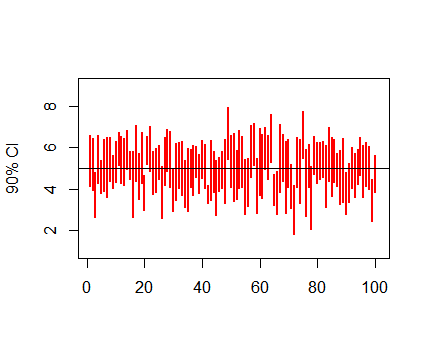实验内容一
1.
1.固定样本量$n = 100$和$\alpha = 0.05$,观察重复次数100、200和400时置信区间包含真值$\mu = 15$的频率是否接近置信度$1- \alpha = 0.95$
# k = 100
CI_L<-NULL
CI_U<-NULL
for (k in 1:100){
samps<-rnorm(100,mean=15,sd=2)
mu<-mean(samps)
sd<-sd(samps)
critv<-qt(1-0.05/2,99)
CI_L<-c(CI_L,mu-critv*sd/sqrt(100))
CI_U<-c(CI_U,mu+critv*sd/sqrt(100))
}
plot(0:100,
type='n',
ylim =c(round(min(CI_L))-1,round(max(CI_U))+1),
xlab ='',
ylab ='95% CI')
segments(1:100,CI_L,1:100,CI_U,col='red',lwd=2)
abline(h=15)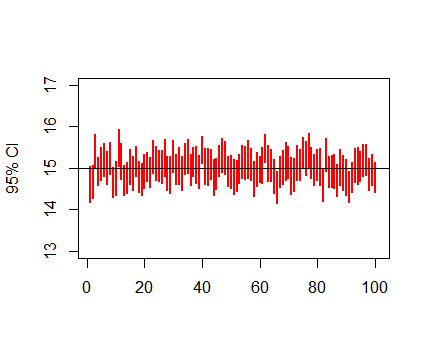# k = 200
CI_L<-NULL
CI_U<-NULL
for (k in 1:200){
samps<-rnorm(100,mean=15,sd=2)
mu<-mean(samps)
sd<-sd(samps)
critv<-qt(1-0.05/2,99)
CI_L<-c(CI_L,mu-critv*sd/sqrt(100))
CI_U<-c(CI_U,mu+critv*sd/sqrt(100))
}
plot(0:200,
type='n',
ylim =c(round(min(CI_L))-1,round(max(CI_U))+1),
xlab ='',
ylab ='95% CI')
segments(1:200,CI_L,1:200,CI_U,col='red',lwd=2)
abline(h=15)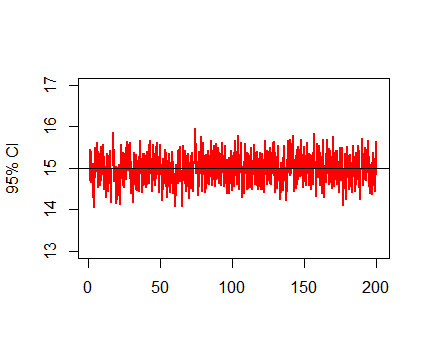# k = 400
CI_L<-NULL
CI_U<-NULL
for (k in 1:400){
samps<-rnorm(100,mean=15,sd=2)
mu<-mean(samps)
sd<-sd(samps)
critv<-qt(1-0.05/2,99)
CI_L<-c(CI_L,mu-critv*sd/sqrt(100))
CI_U<-c(CI_U,mu+critv*sd/sqrt(100))
}
plot(0:400,
type='n',
ylim =c(round(min(CI_L))-1,round(max(CI_U))+1),
xlab ='',
ylab ='95% CI')
segments(1:400,CI_L,1:400,CI_U,col='red',lwd=2)
abline(h=15)2.
2.设置$\alpha = 0.10$，其他保持1不变，重复1，观察模拟结果；并观察与1中置信区间长度对比效果（随的变化）
# k = 100
CI_L<-NULL
CI_U<-NULL
for (k in 1:100){
samps<-rnorm(10,mean=15,sd=2)
mu<-mean(samps)
sd<-sd(samps)
critv<-qt(1-0.1/2,90)
CI_L<-c(CI_L,mu-critv*sd/sqrt(10))
CI_U<-c(CI_U,mu+critv*sd/sqrt(10))
}
plot(0:100,
type='n',
ylim =c(round(min(CI_L))-1,round(max(CI_U))+1),
xlab ='',
ylab ='90% CI')
segments(1:100,CI_L,1:100,CI_U,col='red',lwd=2)
abline(h=15)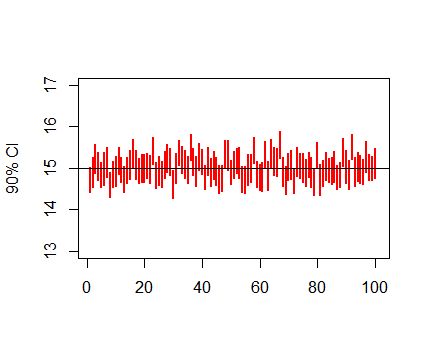# k = 200
CI_L<-NULL
CI_U<-NULL
for (k in 1:200){
samps<-rnorm(100,mean=15,sd=2)
mu<-mean(samps)
sd<-sd(samps)
critv<-qt(1-0.1/2,99)
CI_L<-c(CI_L,mu-critv*sd/sqrt(100))
CI_U<-c(CI_U,mu+critv*sd/sqrt(100))
}
plot(0:200,
type='n',
ylim =c(round(min(CI_L))-1,round(max(CI_U))+1),
xlab ='',
ylab ='90% CI')
segments(1:200,CI_L,1:200,CI_U,col='red',lwd=2)
abline(h=15)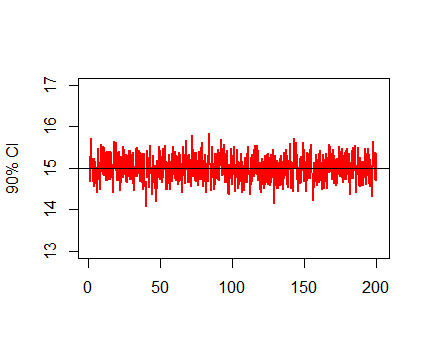# k = 400
CI_L<-NULL
CI_U<-NULL
for (k in 1:400){
samps<-rnorm(10,mean=15,sd=2)
mu<-mean(samps)
sd<-sd(samps)
critv<-qt(1-0.1/2,90)
CI_L<-c(CI_L,mu-critv*sd/sqrt(10))
CI_U<-c(CI_U,mu+critv*sd/sqrt(10))
}
plot(0:400,
type='n',
ylim =c(round(min(CI_L))-1,round(max(CI_U))+1),
xlab ='',
ylab ='90% CI')
segments(1:400,CI_L,1:400,CI_U,col='red',lwd=2)
abline(h=15)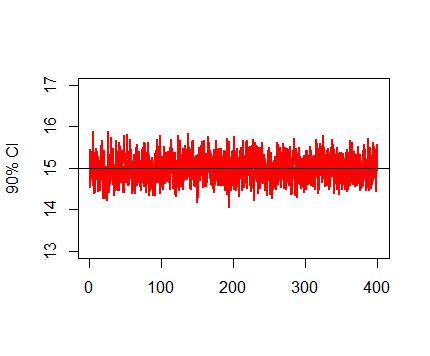3.
3.将1中样本量变成n = 200,其他不变，重复1，观察模拟结果，并观察与1中置信区间长度对比效果（随n的变化）
# k = 100
CI_L<-NULL
CI_U<-NULL
for (k in 1:100){
samps<-rnorm(200,mean=15,sd=2)
mu<-mean(samps)
sd<-sd(samps)
critv<-qt(1-0.05/2,199)
CI_L<-c(CI_L,mu-critv*sd/sqrt(200))
CI_U<-c(CI_U,mu+critv*sd/sqrt(200))
}
plot(0:100,
type='n',
ylim =c(round(min(CI_L))-1,round(max(CI_U))+1),
xlab ='',
ylab ='95% CI')
segments(1:100,CI_L,1:100,CI_U,col='red',lwd=2)
abline(h=15)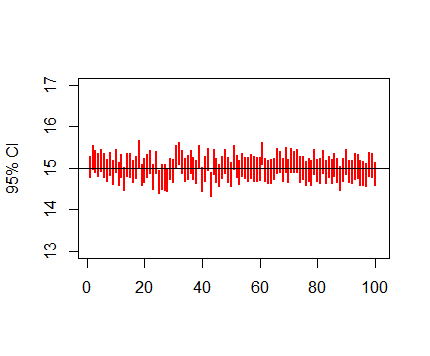# k = 200
CI_L<-NULL
CI_U<-NULL
for (k in 1:200){
samps<-rnorm(200,mean=15,sd=2)
mu<-mean(samps)
sd<-sd(samps)
critv<-qt(1-0.05/2,199)
CI_L<-c(CI_L,mu-critv*sd/sqrt(200))
CI_U<-c(CI_U,mu+critv*sd/sqrt(200))
}
plot(0:200,
type='n',
ylim =c(round(min(CI_L))-1,round(max(CI_U))+1),
xlab ='',
ylab ='95% CI')
segments(1:200,CI_L,1:200,CI_U,col='red',lwd=2)
abline(h=15)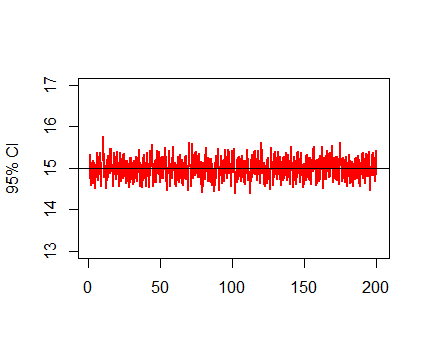# k = 400
CI_L<-NULL
CI_U<-NULL
for (k in 1:400){
samps<-rnorm(200,mean=15,sd=2)
mu<-mean(samps)
sd<-sd(samps)
critv<-qt(1-0.05/2,199)
CI_L<-c(CI_L,mu-critv*sd/sqrt(200))
CI_U<-c(CI_U,mu+critv*sd/sqrt(200))
}
plot(0:400,
type='n',
ylim =c(round(min(CI_L))-1,round(max(CI_U))+1),
xlab ='',
ylab ='95% CI')
segments(1:400,CI_L,1:400,CI_U,col='red',lwd=2)
abline(h=15)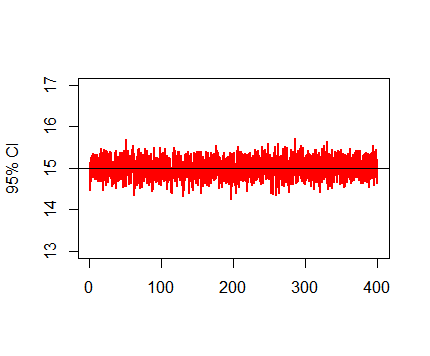展开全文机器学习 算法 聚类算法
• 3、参数估计：区间估计——求置信区间 1）置信区间是什么？ 在样本估计总体均值时，我们需要知道估计的准确度，因此选定一个区间[a,b]，目的是让这个区间包含总体均值，这个区间叫做置信区间。 对于这个区间有...
1、中心极限定理 (Central Limit Theorem)
1）中心极限定理（就是描述样本均值的分布情况）
随着样本容量(Sample size) n趋于无穷，

样本均值(Sampling Distribution of the Sample Mean)的分布越接近正态分布
样本均值的标准差(Standard Error of the Mean) 变小：偏度(Skew)更接近于0，峰度(Kurtosis)也更接近于0
这里样本均值指的是选取多个样本，每个样本可以求出一个样本均值，多个样本均值的分布符合正态分布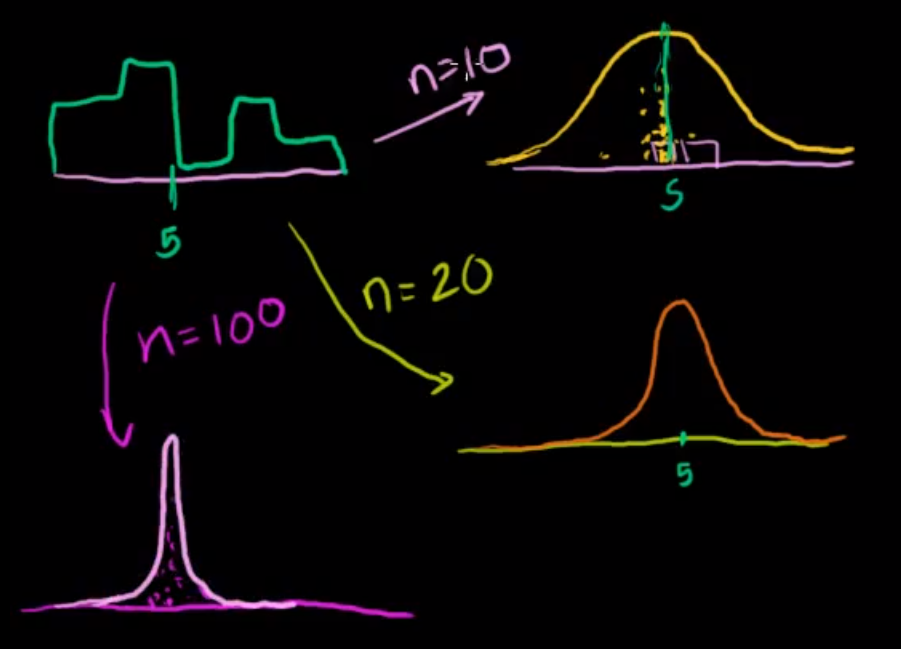大数定律 Law of Large Number：随着样本容量n越大，样本均值越接近总体均值

除了样本均值，样本众数、样本和，样本极差等统计量也适用

一般n>30即可看作样本均值为正态分布

2）样本均值的抽样分布
样本均值的标准差(Standard Error of the Mean)
$\sigma_{\overline{x}}=\frac{\sigma}{\sqrt{n}}$
偏度 (Skew)峰度(Kurtosis)3）利用标准正态分布求概率的方法

确定分布（均值$\mu$和标准差$\sigma$）与范围
标准化，使其均值为0，标准差为1，得出标准正态变量Z，$Z\sim N\left(0,1\right)$
查概率表

标准化是为了使其对应查找标准正态分布概率表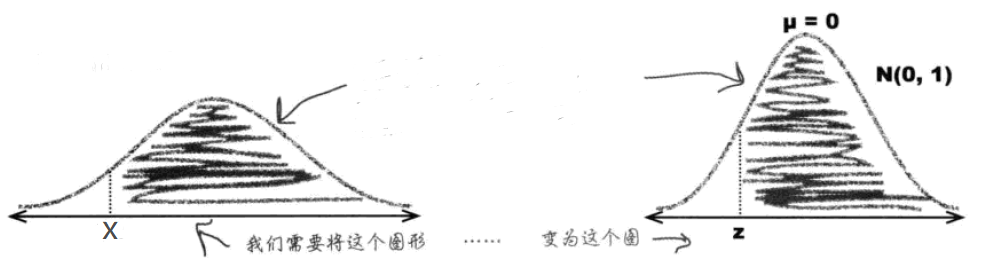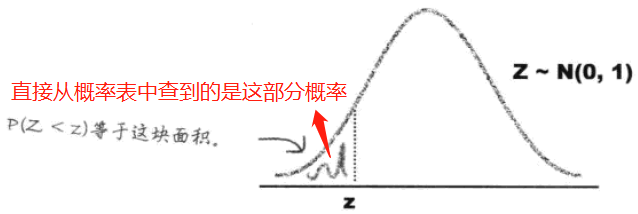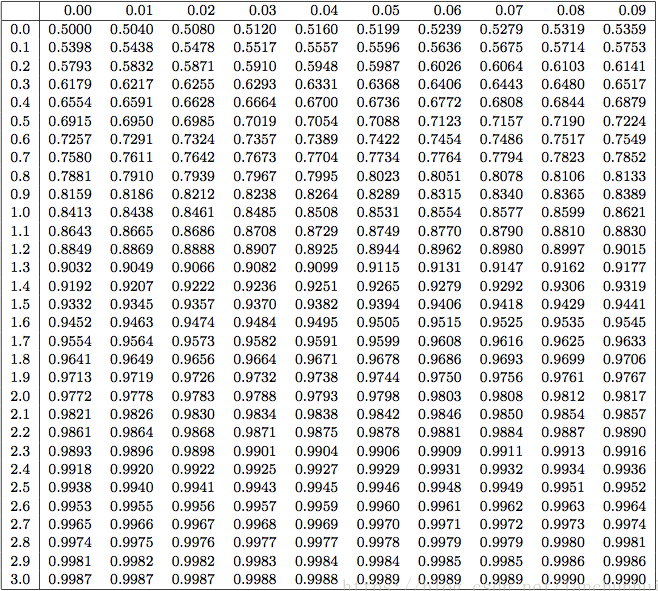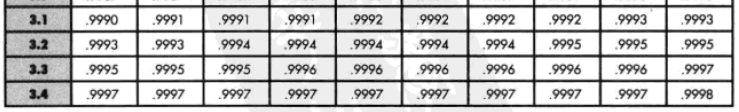4）实例
（1）男性在户外活动时平均喝2L水（标准差是0.7L）。50人全天户外旅行，准备110L水．这些水不够的概率是多少？

确定分布

50个人看作样本，样本容量 $n=50$

50人准备110L水，即平均喝水 $\overline{x}=110/50＝2.2L$，均值分布服从正态分布

求水不够的概率，等价于求平均喝水超过2.2L的概率$P\left(\overline{x}>2.2\right)=?$

样本均值抽样分布的标准差$\sigma_{\overline{x}}=\frac{\sigma}{\sqrt{n}}\approx0.099$

标准化
$z=\frac{\overline{x}-\mu}{\sigma}=\frac{2.2-2}{0.099}\approx 2.02$
查概率表
查表可知$P\left(Z>2.02\right)=1-0.9783=0.0217$2、参数估计：点估计
$\overline{x}：样本均值\\ \mu:总体均值\\\hat\mu:总体均值的点估计$
均值的点估计：:$\hat\mu=\overline{x}$$S^2:样本方差\\\sigma^2:总体方差\\\hat\sigma^2:总体方差的点估计$
方差的点估计（用样本数据估计总体方差）：$\hat\sigma^2=S^2=\frac{\sum_({x-\overline{x}})^2}{n-1}$
2) 区间估计
其实就是求置信区间 见下
3、参数估计：区间估计——求置信区间
1）置信区间是什么？
在样本估计总体均值时，我们需要知道估计的准确度，因此选定一个区间[a,b]，目的是让这个区间包含总体均值，这个区间叫做置信区间。
对于这个区间有多大概率包含总体均值，这个概率称为置信水平，是我们对这个范围的可信程度。。置信水平是根据实际问题自己确定的，一般设定为95%即两个标准差。
2）怎么计算置信区间？（结合例子更具体的讲解可以看深入浅出统计学）
解题的时候要区分清楚哪些是样本统计量（已知），哪些是总体统计量（未知，通过点估计得出）

选择总体统计量
即确定你要求的那个总体均值 $\mu$
求样本均值的抽样分布
1)计算样本均值 $\overline{x}$
2)计算样本均值标准差 $\sigma_{\overline{x}}=\frac{\sigma}{\sqrt n}\approx \frac{S}{\sqrt n}$
（由于事先我们并不知道总体的标准差。因此要用样本方差作为总体方差的估计（点估计），需注意是会跟随样本的变化而变化。）
3)得出样本均值的分布：$\overline{X}\sim N\left(\overline{x},\sigma_{\overline{x}}\right)$，因为这里就是通过样本均值估计总体均值的区间，所以把$\overline{x}$换成$\mu$，$\overline{X}\sim N\left(\mu,\sigma_{\overline{x}}\right)$
确定置信水平
求出置信上下限
1)当样本容量较大（>30）时，查找z表格；
a. 标准化
即把$\overline{X}\sim N\left(\mu,\sigma_{\overline{x}}\right)$转换成标准正态分布$\overline{Z}\sim N\left(0,1\right)$，Z就是标准化后的$\overline{X}$ $Z=\frac{\overline{x}-\mu}{\sigma_{\overline{x}}}$
b. 用$\mu$改写不等式
（以置信水平为95%为例）
$P(-1.96<Z<1.96)=0.95\\P(-1.96<\frac{\overline{x}-\mu}{\sigma_{\overline{x}}}<1.96)=0.95$
其中$\overline{x}、\sigma_{\overline{x}}$已知，带入求出$a<\mu<b$即可得出置信区间(a,b)

置信区间简便算法，用下面的表可以取代第4步，直接带入求出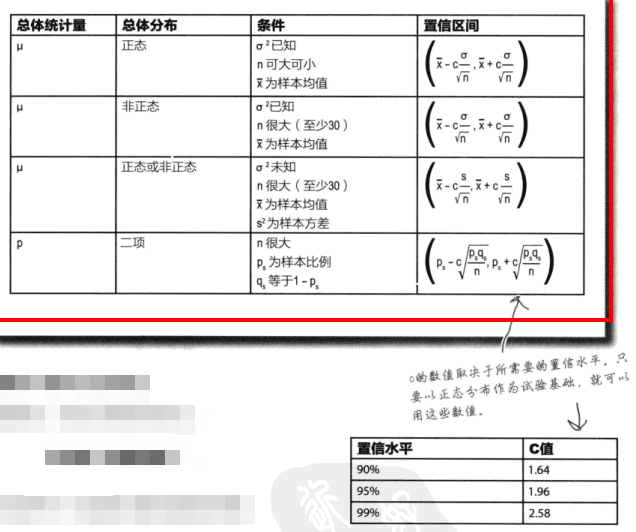2)当样本容量较小（<30）时，为t分布，确定自由度(degrees of freedom) t=n-1，查找t分布表（跟正态分布的计算差别只在查表，其他都相同）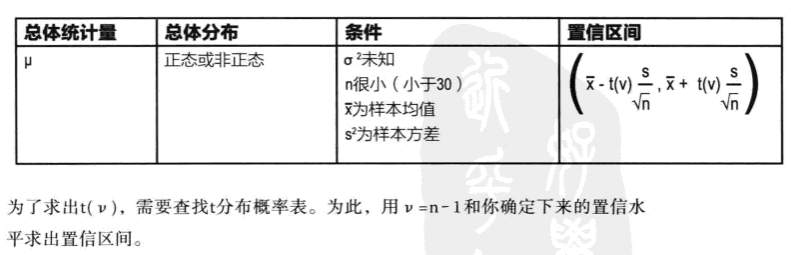3）实例
(1) 某地区教学区获得一批技术拨款，用于在教师中安排4台一组的计算机．该区总共有6250名教师，随机抽取250名，并且问他们是否认为计算机是教师必备的教学工具．抽取的教师中，有142名认为计算机是教学必备的工具．
问题1：
计算一个99％置信区间，其中教师认为计算机是必备的教学工具．
定义：
1表示计算机被认为是必备工具，占比为p，
0表示计算机被认为不是必备工具，占比为q=1-p．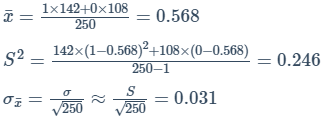z表格的值应该为0.99/2+0.5＝0.995
对应2.58个标准差处
$0.568\pm2.58\times0.031 = 0.568\pm0.08$
即 $0.488\sim0.648=48.8\%\sim64.8\%$
有99%的几率，48.8%~64.8%的老师认为计算机是必备的
问题2：
保持99％置信水平的前提下，如何缩小置信区间？
抽取更大的样本.
(2) 小样本容量置信区间
7个患者在服用新药3个月后测量血压．其血压上升值分别为1.5, 2.9, 0.9, 3.9, 3.2, 2.1, 1.9．为总体中所有病人的血压升高真正期望值建立一个95％的置信区间。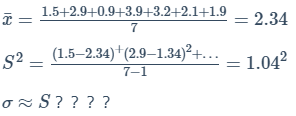这里样本容量太小了，不能认为其样本均值为正态分布，不能使用中心极限理论。可以认为是t分布，查t分布表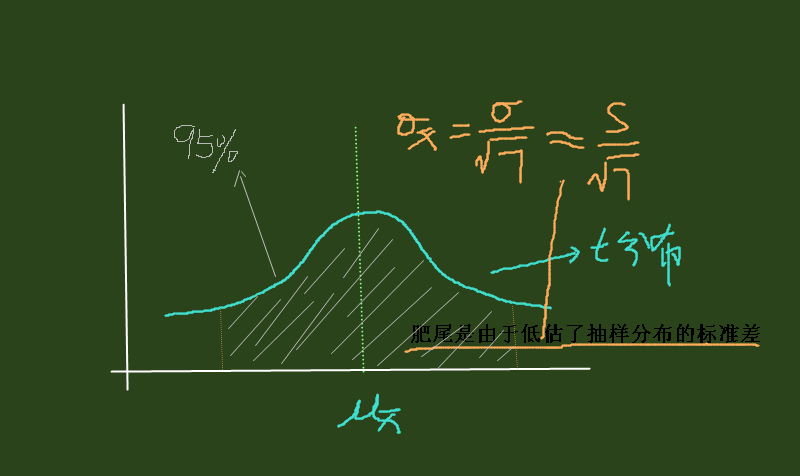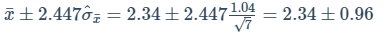即 $1.38\sim3.3$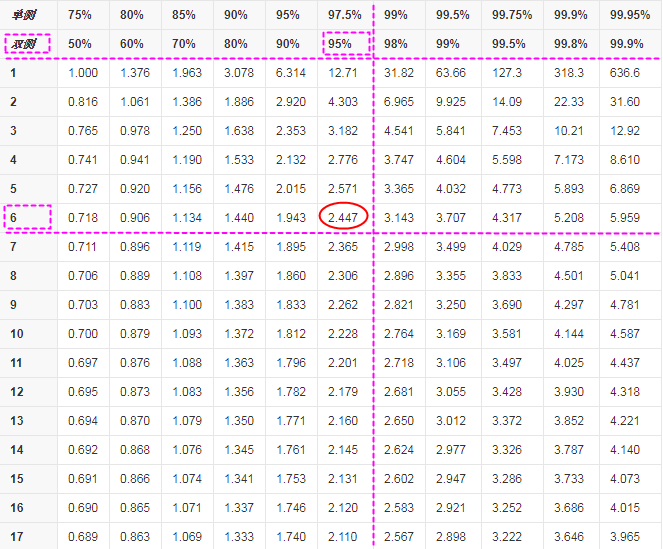参考资料：
可汗学院统计学：https://www.bilibili.com/video/av7199273/?p=73
简客：https://jentchang.github.io/contents/math/statistical.html
《深入浅出统计学》


展开全文统计学 数据分析
• 置信区间估计 预测区间估计Estimation implies finding the optimal parameter using historical data whereas prediction uses the data to compute the random value of the unseen data. 估计意味着使用历史数据...
置信区间估计 预测区间估计Estimation implies finding the optimal parameter using historical data whereas prediction uses the data to compute the random value of the unseen data. 估计意味着使用历史数据找到最佳参数，而预测则使用该数据来计算未见数据的随机值 。
The highlighted words in the above statement need some context setting before we proceed further: 在继续进行之前，上述语句中突出显示的词需要进行一些上下文设置：
We need lot of historical data to learn dependencies for machine learning and modelling. The data typically involves multiple observations, where each observation consists of multiple variables. This multivariate observation x belongs to random variable X whose distribution lies in the realm of a finite set of possible distributions called as ‘the states of nature’. 我们需要大量的历史数据来学习机器学习和建模的依赖关系。 数据通常包含多个观察值，其中每个观察值都包含多个变量。 该多元观测值x属于随机变量X，其分布位于称为“自然状态”的有限分布的可能范围内。 Estimation is the process of optimizing the true state of nature. Loosely speaking, estimation is related to model building i.e. finding the most appropriate parameter that best describes the multivariate distribution of historical data, for e.g. if we have five independent variables, X1, X2….X5 and Y as the target variable. Then, estimation involves the process of finding f(x) which is the closest approximation of the true state of nature denoted by g(θ). 估计是优化自然真实状态的过程 。 宽松地说，估计与模型构建有关，即找到最能描述历史数据多元分布的最合适参数，例如，如果我们有五个独立变量X1，X2….X5和Y作为目标变量。 然后，估计涉及寻找f(x)的过程，f(x)是由g(θ)表示的真实自然状态的最近似值。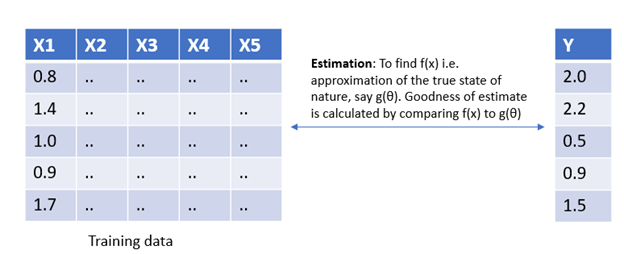Parameter estimation on training dataWhereas, prediction leverages the already built model to compute the out of sample values. It is a process of calculating the value of another random variable Z whose distribution is related to the true state of the nature (this property plays a pivotal role in any machine learning algorithm). Predictions are considered good when they agree over all the possible values of Z, on an average. 而预测则利用已经建立的模型来计算样本外值。 这是计算另一个随机变量Z的值的过程，该变量的分布与自然的真实状态有关(此属性在任何机器学习算法中都起着关键作用)。 平均而言，当预测与Z的所有可能值一致时，这些预测就被认为是好的。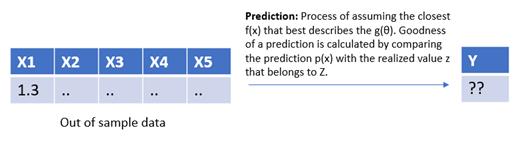Prediction on unseen dataThere are multiple ways to interpret the difference between the two, let’s also explore the Bayesian intuition: 解释两者之间差异的方法有多种，让我们还探讨贝叶斯直觉 ：
Estimation is after the occurrence of the event i.e. posterior probability. Prediction is a kind of estimation before the occurrence of the event i.e. apriori probability. 估计是在事件发生之后，即后验概率。 预测是在事件发生之前进行的一种估计，即先验概率。
Let’s summarize our understanding on estimation and prediction: To make predictions on unseen data, we fit a model on training dataset that learns an estimator f(x), which is used to make predictions on new data. 让我们总结一下对估计和预测的理解：为了对看不见的数据进行预测，我们在训练数据集上拟合了一个模型，该模型学习了估计器f(x)，该函数用于对新数据进行预测。
Now, that we understand what the prediction is, let’s see how it is different from forecasting. 现在，我们了解了预测是什么，让我们看看它与预测有何不同。
Forecasting problems are a subset of prediction problems wherein both use the historical data and talk about the future events. The only difference between forecasting and prediction is the explicit addition of temporal dimension in forecasting. 预测问题是预测问题的子集，其中既使用历史数据，又谈论未来事件。 预测与预测之间的唯一区别是在预测中显式增加了时间维度。
Forecast is a time-based prediction i.e. it is more appropriate while dealing with time series data. Prediction, on the other hand, need not be time based only, it can be based on multiple causal factors that influence the target variable. 预测是基于时间的预测，即在处理时间序列数据时更合适。 另一方面，预测不必仅基于时间，它可以基于影响目标变量的多个因果因素。
I stumbled across a very fresh perspective of explaining the difference between the prediction and forecast using the analogy of the origin of the words themselves. 我偶然发现了一个非常新颖的观点，即使用单词本身的起源来解释预测与预测之间的差异。
I will brief on this innovative illustration in this post, but you can read more about it at the original post here. 我将在这篇文章中简要介绍这个创新的插图，但是您可以在此处的原始文章中了解更多有关它的信息。
Forecast is more process-oriented and follows a certain methodology of doing something. In a way, it assumes that the past behavior is a good enough indicator of what is going to happen in the future. 预测更注重过程，并遵循某种方法进行工作。 在某种程度上，它假设过去的行为足以说明将来会发生什么。
Prediction considers all historical processes, influencing variables and interactions to reveal the future. 预测考虑了所有历史过程，影响变量和相互作用以揭示未来。
In summary, all forecasts are predictions but not all predictions are forecasts. 总之，所有预测都是预测，但并非所有预测都是预测。 Hope you now have clarity on the difference between estimation and prediction. The post also highlights the distinction between prediction vs forecast. 希望您现在对估计和预测之间的区别有所了解。 该帖子还强调了预测与预测之间的区别。
References: https://stats.stackexchange.com/questions/17773/what-is-the-difference-between-estimation-and-prediction/17789#17789 参考： https : //stats.stackexchange.com/questions/17773/what-is-the-difference-between-estimation-and-prediction/17789#17789
翻译自: https://towardsdatascience.com/estimation-prediction-and-forecasting-40c56a5be0c9置信区间估计 预测区间估计
展开全文机器学习 人工智能 python 算法 深度学习
• 尽力搞懂统计学概念——点估计、区间估计置信区间 在统计学的应用过程中，总有那么几个重要的基础概念似懂非懂，就像雾里看花，你对它有一个总体的印象，但说道具体细节又似是而非。我也深受其扰，现在就结合...
文章目录点估计区间估计置信区间理解操作（重中之重）总体方差已知总体方差未知假设检验

在统计学的应用过程中，总有那么几个重要的基础概念似懂非懂，就像雾里看花，你对它有一个总体的印象，但说道具体细节又似是而非。我也深受其扰，现在就结合自己的思考和网上各路大神的指点，试着通俗易懂地论述一下。

在开始之前先说一下统计学中的几个基本概念的定义，有助于后面的理解。

总体（population）：是指研究对象的某个数量指标的全体。
重点是“某个数量指标”，比如研究某个城市所有人的身高，则总体是这个城市内所有人的身高，而不是所有人。
一般来说把总体和随机变量X等同起来。

样本（sample）：
n个相互独立且与总体X同分布的随机变量X1,X2...,Xn的整体(X1,X2,...,Xn)成来自总体 X 的容量为n的一个样本。一次抽样结果的n个值被称为样本的一个观测值或者说是样本值。

统计量：
X1,X2,...,Xn是来自总体X的样本，g(X1,X2,...,Xn)是n元函数，如果g中不包含未知参数，则称g(X1,X2,...,Xn)是样本X1,X2,...,Xn的一个统计量。
关键点是“不含未知参数”，是“完全取决于样本的量”，说人话就是 样本均值、样本方差等就是所谓的统计量。

点估计
很简单，点估计就是用一个样本统计量来估计一个总体未知参数。
例如总体X是一个公司所有10,000名员工的年薪，目标是估计所有员工的平均年薪。点估计就是采用简单随机抽样的方法随机抽取1000名员工并统计他们的年薪，计算出1000人的平均年薪，以此来估计总体均值。当然这样是很不准的，甚至从总体X的概率密度函数上来说  P(总体均值μ-样本均值=0)=0。
区间估计
区间估计就是在点估计的基础上，给点估计值加上左右领域，给出总体参数估计的一个区间范围。
先这样简单理解一下，具体会在下面深度说明。
置信区间
理解
试想一下，我们是某大厂的员工，在职员工10,000人。
想知道自己的年薪在整个公司处于上游还是下游，但对于整个公司所有人的平均薪资我们是不知道的。
但这个总体均值μ是客观存在的，只是我们作为一名小员工不知道，我们可以认为，HR知道，假设总体的年薪服从正态分布。
HR内心OS：我知道今年所有人的平均年薪μ=100k,方差σ^2=2，也就是总体 X ~ N（100,2）,如下图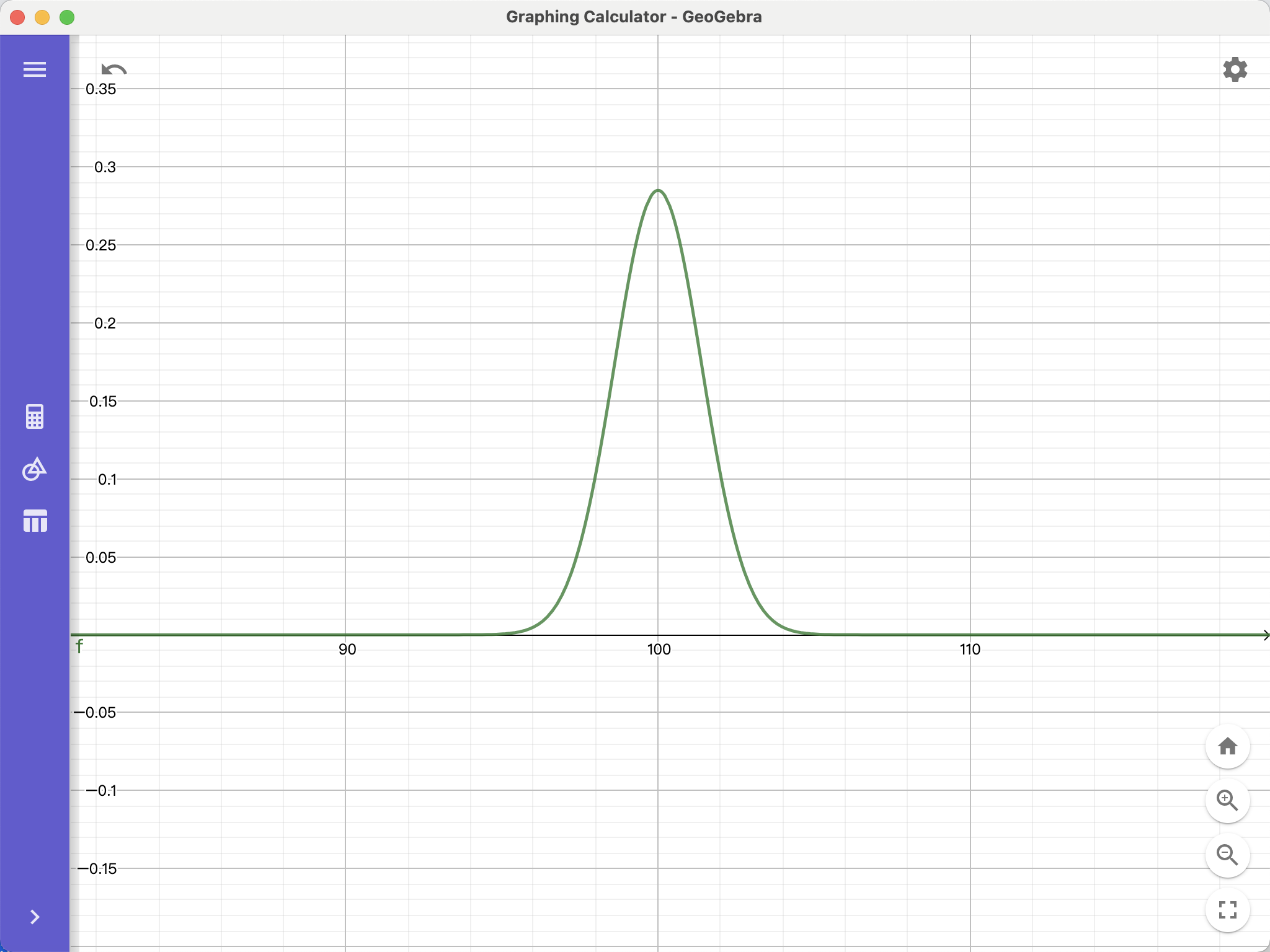我们不可能一个一个人问过来，这样大概率也会被叫去谈话。所以随机抽取样本容量为100的同事的年薪，并计算出样本均值假设是15k，这就是点估计。
但你会想：明明我只有8k啊，为什么有15k，你觉得不准，于是经过一次又一次地抽样，得出以下的结果：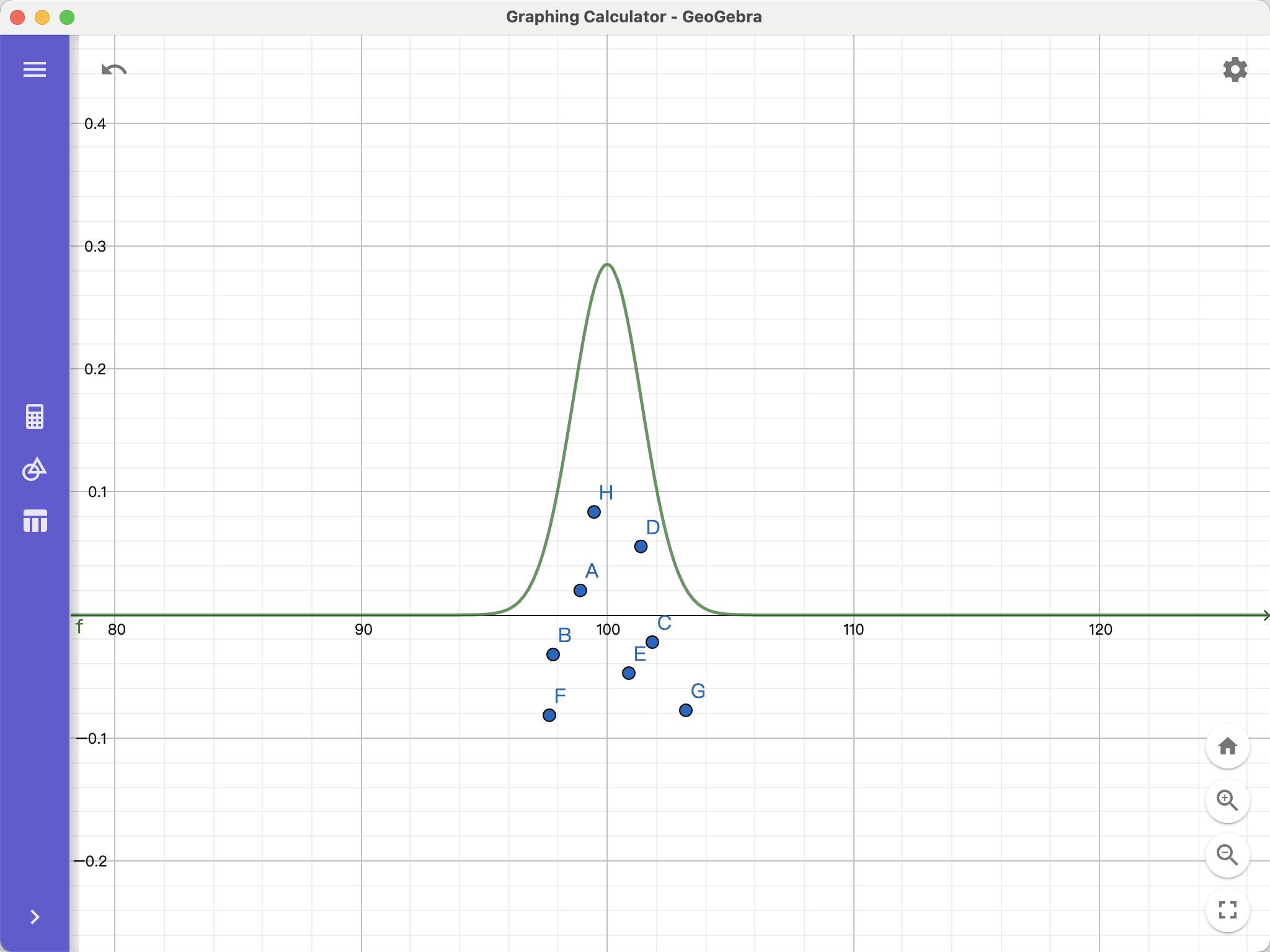那哪个点估计更好呢？？😟😟😟咋办
于是我们采用区间估计来改善这个问题。

在95%的置信区间上,针对每个点估计构造区间估计。在HR视角下，可以发现大部分的区间估计都包含了总体均值，只有点G这一条没有包含。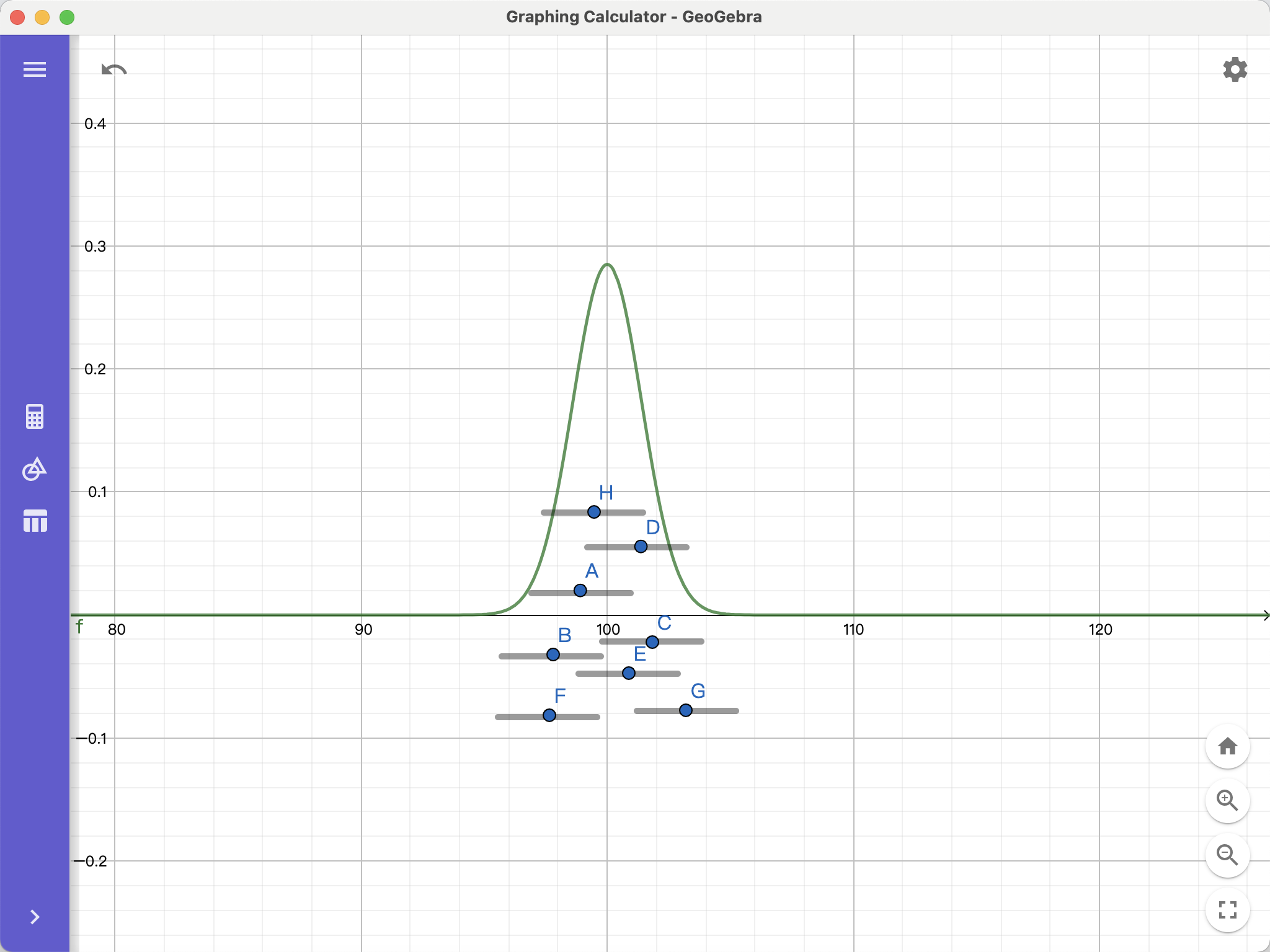但是失去了HR视角，我们还是不知道哪个区间估计更好，要是你采用了点G的区间估计，甚至不知道自己估错了。

是的，无论是点估计还是区间估计，我们都无法知道哪个点或者那个区间估计的更好，但是在95%的置信度下构造的区间估计，我们可以说，如果构造100个区间估计，那大概有95个是包含真实的总体均值的。
操作（重中之重）

以上是对区间估计的概念理解，在实际情况下，我们当然不会去构造100个区间估计。而是通过一次抽样得到的样本均值，设定显著性水平α，在 1-α 的置信区间内给出基于这个样本均值的区间估计。

接下来是数学推导
一些公式打起来不方便 就手写了
总体方差已知
设正态总体 X~N(μ,σ^2)，样本X1,X2…,Xn独立同分布于总体X，
由样本均值x的期望 E(样本均值x)=E[1/n(X1+X2+...+Xn)]=1/n * nμ=μ
方差 D(样本均值x)=D[1/n(X1+X2+...+Xn)]=1/n^2 * n * σ^2= σ ^2/n
将其标准化=> (样本均值x-μ)/(σ/√n) ~ N(0,1)
我们想要在α=0.05的显著性水平，即1-α=95%的置信区间下对总体均值μ做区间估计。
用概率表示出来是 P( |样本均值x-μ|<△)=1-α
不等号的左右恒等变形 P( |(样本均值x-μ)/(σ/√n)|<△/(σ/√n))=1-α ①
根据上面的推导，①左边服从于标准正态分布，如下图：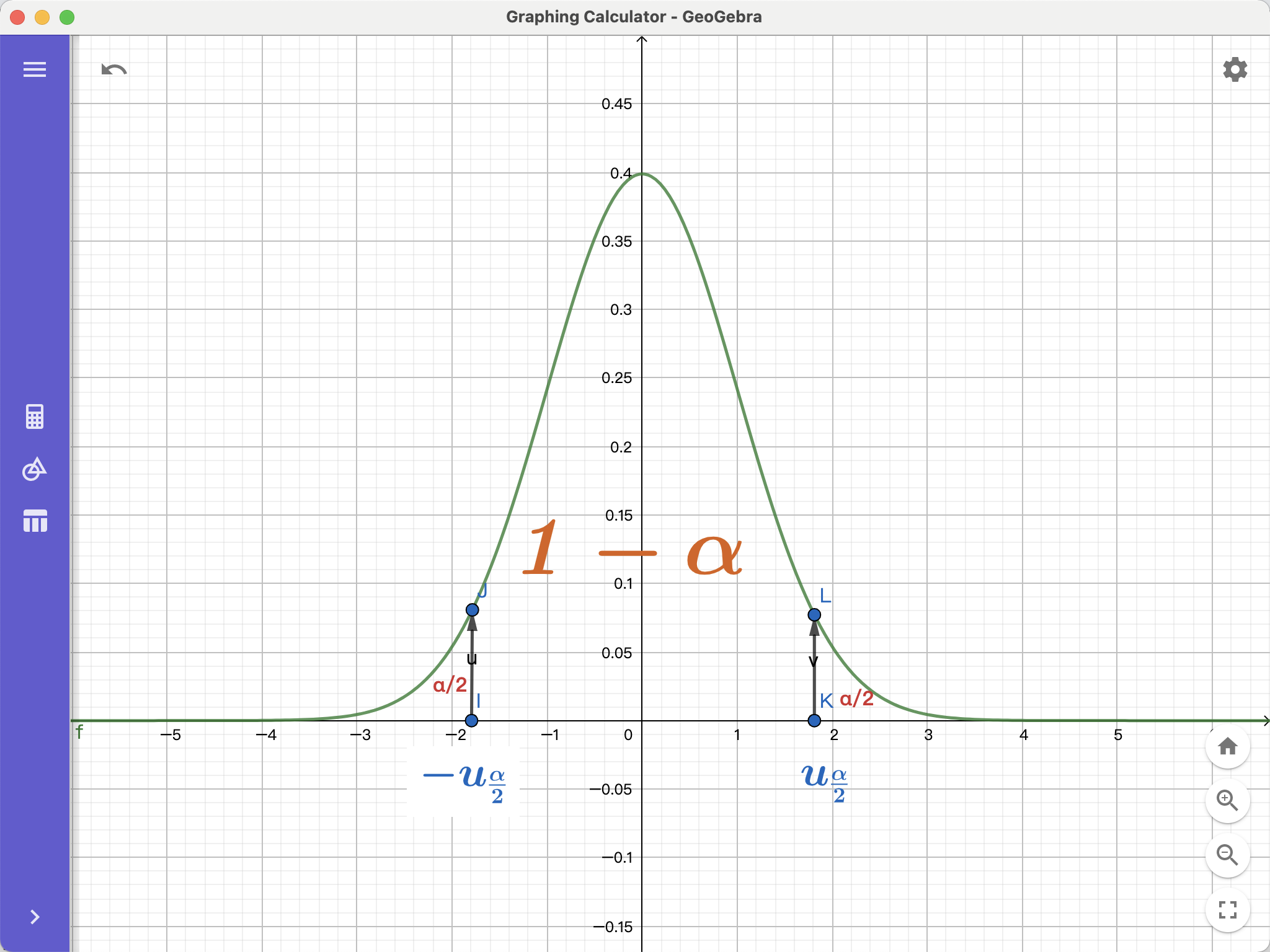1-α是中间那块区域，两边的面积都等于α/2，
点K的横坐标可以用标准正态分布分位数 uα/2 表示（α=0.05）
这是一个实际已知的数，可以通过查标准正态分布分位数表获得。
所以 △= uα/2*(σ/√n)
=> P(|样本均值x-μ|<uα/2 * (σ/√n))=1-α
将式子解出来，可得
样本均值x - uα/2 * (σ/√n)<μ<样本均值x+uα/2 * (σ/√n)
总体方差未知
在现实情况中，我们往往不知道总体方差（除了HR）
那总体方差未知时应该怎么办呢😰
这时候就要用样本方差 s^2 代替总体方差 σ^2。
这里先简单介绍一下两个分布：卡方分布和t分布。

卡方分布：
随机变量X1,X2...,Xn 独立同分布于标准正态分布 N(0,1)，则
(X1 ^2 + X2 ^2 +...+Xn ^2 )服从于自由度为n的卡方分布
t分布：
随机变量 X 服从于标准正态分布，随机变量 Y 服从于自由度为n的卡方分布，且 X与Y 相互独立，则随机变量 t = X/二次根号(Y/n) 服从于自由度为 n 的 t分布。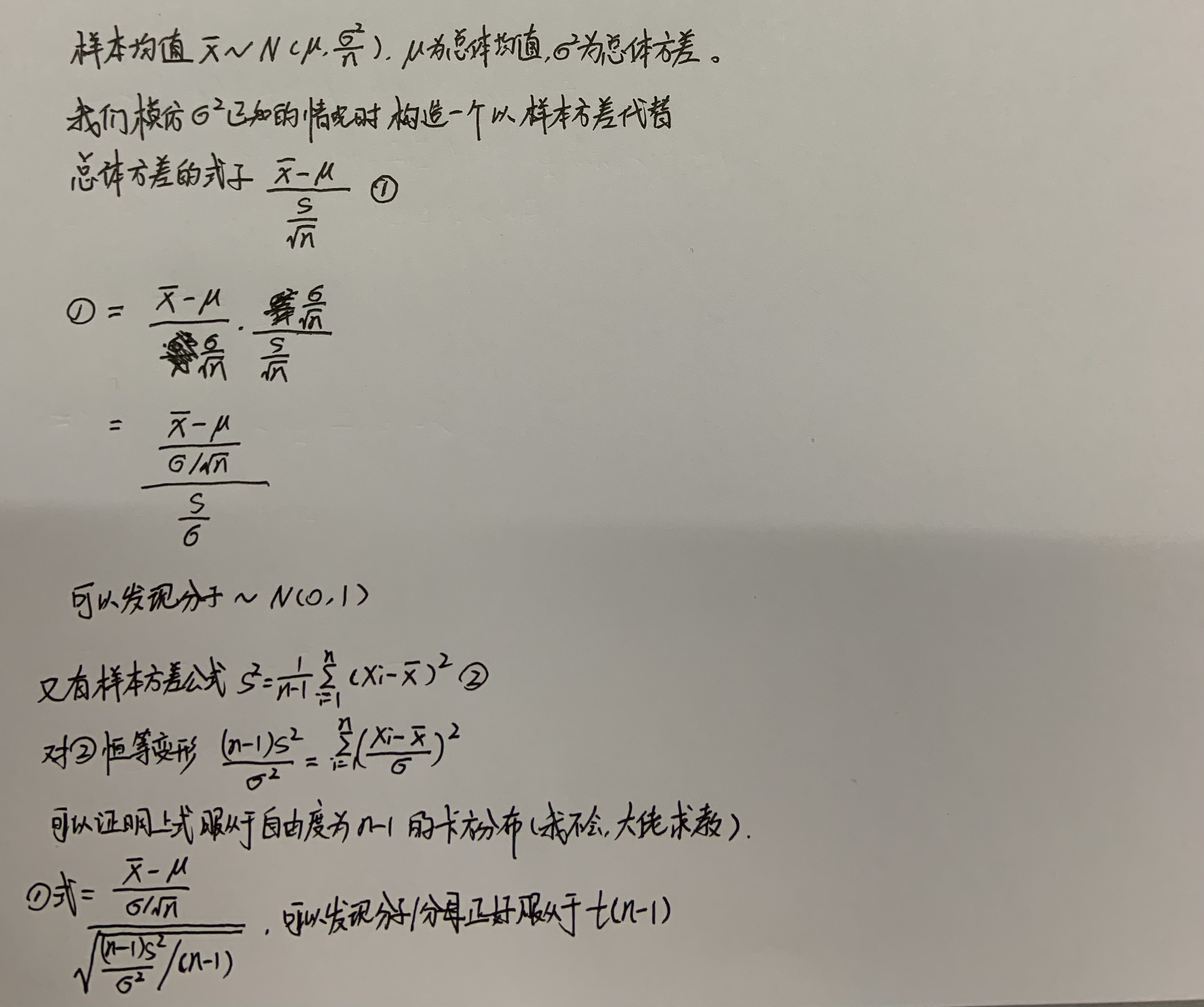t分布的概率密度函数图像与标准正态分布的形似，自由度n越小，则曲线越平坦，自由度n越大，曲线越接近标准正态分布，当自由度趋于+∞时，t分布就是标准正态分布。
所以在用样本方差代替总体方差做区间估计的情况下，其推导方法与用总体方差时一致，不过把标准正态分布换成了自由度为 n-1 的t分布。
所以相应的，式子
P( |总体均值μ-样本均值| < △) = 1-α
此时用t(n-1)分位数代替标准正太分位数，
△ = t(n-1)α/2分位数 * s/二次根号(n)，
总体均值的区间估计为 （μ - △ , μ + △）。
假设检验
累了，下次再讲


展开全文• 一种基于Bootstrap的Web服务QoS置信区间估计和预测方法研究论文
• 什么是置信区间和置信水平？ 置信区间？ 95%置信区间应该这样理解：做100次相同的抽样，计算置信区间，那么你计算的区间内包含整体均值的概率是95%。置信区间描述的是随机抽样的可信度，说白了就是证明你抽样给出的...
• 在统计学中，一个概率样本的置信区间是对这个样本的某个总体参数的区间估计置信区间展现的是这个参数的真实值有一定概率落在测量结果的周围的程度，其给出的是被测量参数的测量值的可信程度，即前面所要求的“一个...
• 什么是预测区间和置信区间 最近需要画带有置信区间的拟合图，其中在matlab的doc中搜索“Confidence and Prediction Bounds”，出现了两种置信区间。 原博客：https://www.cnblogs.com/100thMountain/p/5539024.html ...
• 有两种形式：点估计和区间估计 （2）假设检验问题：总体X的分布函数的形式完全未知，或只知其形式，但不知其参数，为了推断总体的某些未知特性，提出某些关于总体的假设。 区间估计 引入：点估计值仅仅是未知参数的...机器学习
• 指定 interval level 参数时， predict.lm 可以返回置信区间(CI)或预测区间(PI) . 此答案显示如何在不设置这些参数的情况下获取CIPI . 有两种方法：使用 predict.lm 的中期结果;从头开始做一切 .了解如何使用这...
• ## 置信度和置信区间

千次阅读 2019-06-11 15:50:02
置信度：也称可靠度，置信水平，即估计值与总体参数在一定允许的误差...置信区间置信区间是由样本（总体样本，不是真实样本）构造的对总体参数估计的一个区间，每一个置信区间会对应一个置信水平，表示真实参数落...概率论与数理统计 深度学习
• 数理统计——点估计置信区间一、样本估计总体总体均值的估计总体方差的估计二、总体估计样本(样本Xs∼B(n,p)X_{s}\sim{B(n,p)}Xs​∼B(n,p))三、总体估计样本均值(已知μ\muμσ2\sigma^2σ2) 一、样本估计总体 ...
• 基于此,提出一种置信区间检验方法,利用残差、均方根误差和置信概率作为参考值,选择最佳的初始种子点。采用格网重叠方式解决相邻格网间板块化问题,同时应用分层聚类自适应阈值确定方法确定高差阈值。对特殊种子点不足...
• 二、置信区间的求法问题:设总体X的分布有未知参数 是一样本.如何给出θ的(1) 置信水平为...因为要求的区间估计，所以G应该是 样本 的函数.(3)从 解出 , 就是置信度为的双侧置信区间. 设总体 有概率密度（或分布律...
• 有不少小伙伴在后台留言希望多讲讲假设检验和置信区间的内容，在此对同学们的关注表示感谢，同时，我们也会尽力加快推文速度。首先，简单回顾一下，抽样分布是针对样本统计量而言的，比如样本均数。在同一个总体中，...
• 1.误差和置信区间的关系在测量、计算和观察过程中由于错误或者不可控因素的影响造成的变化偏离标准值或者规定值，这种误差是不可避免的，只要有估计，就会有误差，面对这种情况，我们会给予一个误差范围，在统计学中...
• 1.大样本如何计算置信区间(z统计量）确定要求解的问题：根据样本估计全国男性身高的均值求样本的平均值标准差样本大小是100人，样本平均值是167.1cm,样本标准差是0.2cm标准误差:0.02cm确定置信水平：常用的置信...
• 在这种情况下，统计学家提出了一种称为t分布来对均值的置信区间进行估计。通过以下例子可以让我们对均值的置信区间估计有更深了解。在纽约州，存储性银行被允许销售一种称为储蓄银行寿险(SBLI)的保险产品。保险业的...标准差σ未知
• Interval] -------------+--------------------------------------------------------------- | 12 3.816667 .0782409 3.64446 3.988874 大家好，请问为什么ci做出来的group1 x1的置信区间和cii做出来的不一样......